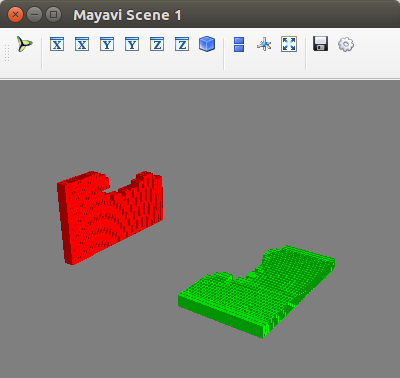# Image Sampler 3D

Hey everyone,
since I’m dealing with 3D (depth, height, width) data, I’m wondering if someone has implemented a fast image warping function in PyTorch. I’m aware of the grid_sample function, but so far, it only supports two dimensional data. If anyone has some hints where to look, please let me know! Or if anyone from the core team could provide some information on wether the expansion to 3D is planned in the near future, that would be great!

The function is implemented at it’s core in these two files:

It is a relatively small task to extend this for 3D inputs. One has to make VolumetricGridSamplerBilinear.c / cu and call them from here: https://github.com/pytorch/pytorch/blob/1c0fbd27a10b7036b1db643014e65cae7f6d0266/torch/nn/_functions/vision.py#L52

Thank you for this fast reply!
Since I’m not familiar with writing code in cuda, this will surely be a hard task for me, but I’ll try my bestThe way the code is written, you only have to add an additional for loop everywhere (even in CUDA case), so it shouldn’t need any working knowledge of cudaAwesome. I’ll give it a shotIs there any chance that this will be implemented in the soon future?
If it’s a relatively small task, then why not implement it in the core?`grid_sample` supports 3D since February, and release `0.4.1` supports this feature:

1 Like

Hi @smth I am trying to use `grid_sample` to implement stn for 3d data. Here is a simple test code

``````import torch

def create_grid(height=32, width=32, depth=32):
# create normalized 2D grid
x = torch.linspace(-1, 1, width)
y = torch.linspace(-1, 1, height)
z = torch.linspace(-1, 1, depth)
x_t, y_t, z_t = torch.meshgrid([x, y, z])

# flatten
x_t_flat = x_t.contiguous().view(-1)
y_t_flat = y_t.contiguous().view(-1)
z_t_flat = z_t.contiguous().view(-1)

# reshape to [x_t, y_t , 1] - (homogeneous form)
ones = torch.ones_like(x_t_flat)
sampling_grid = torch.stack((x_t_flat, y_t_flat, z_t_flat, ones), 0)

# repeat grid num_batch times
print(sampling_grid.shape)
sampling_grid = sampling_grid.unsqueeze(0)
print(sampling_grid.shape)
return sampling_grid

def stn(grid, sampling_grid, theta, grid_size):
# grab batch size
num_batch = theta.shape
sampling_grid = sampling_grid.repeat(num_batch, 1, 1)
sampling_grid_t = torch.matmul(theta, sampling_grid) # B*3*HWD
sampling_grid_t = sampling_grid_t.permute([0,2,1]) # B*HWD*3

# sampling_grid_t = torch.tensor(sampling_grid_t.numpy().reshape(num_batch, grid_size, grid_size, grid_size, 3))
sampling_grid_t = sampling_grid_t.view(num_batch, grid_size, grid_size, grid_size, 3)
out = torch.nn.functional.grid_sample(grid, sampling_grid_t)
return out
``````

But when i pass the `stn` a 3d grid with identity transformation

``````theta = torch.cat((torch.eye(3),torch.zeros(3,1)), 1)
theta = theta.unsqueeze(0)
``````

I got a rotated grid for some reason as shown in the picture below. I suspect it’s a problem with the way the sampling grid is defined. but there are no details in the documentation about how to do this in 3d. Can you please help?2 Likes

were you able to figure this out?

How did you plot this? is your data or what the grid_sampler is doing?